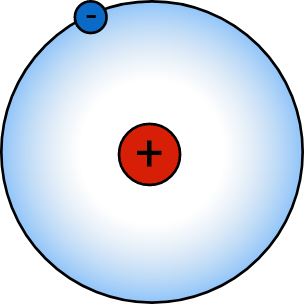# Very Small Physics 2Hydrogen is the simplest atom, with only one proton and one electron interacting with a Coulomb potential of $U = -\frac{e^2}{4 \pi \varepsilon_0 r}.$ If the electron were a classical charged particle, its orbit around the nucleus would decay until it came to rest at the nucleus. Since the electron is a quantum object, this doesn't happen. One way to estimate the minimum radius allowed for a quantum electron is by saturating the Heisenberg bound, which puts a lower limit on the radius of the electron's orbit $r$ and its momentum $p$: $r p \approx \Delta x \Delta p \approx \hbar.$ Treat the hydrogen atom as a two-body problem where the mass of the proton $m_p$ is much greater than that of the electron $m_e.$

Estimate the minimum radius of the electron orbit $r_\text{min}.$

Details: $e$ is the charge of an electron, $\varepsilon_0$ is the permittivity of free space, and $\hbar$ is the reduced Planck's constant.

×